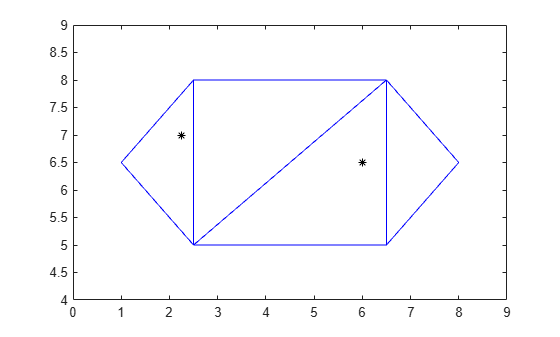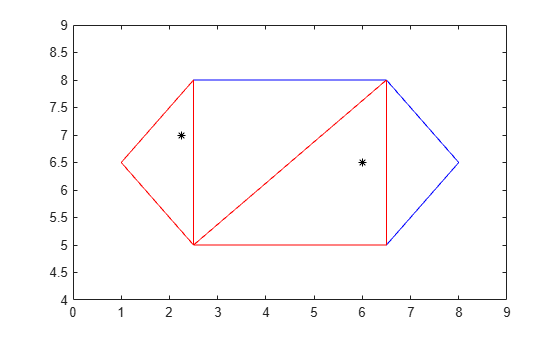# pointLocation

Triangle or tetrahedron enclosing point

## Syntax

``ID = pointLocation(TR,P)``
``ID = pointLocation(TR,x,y)``
``ID = pointLocation(TR,x,y,z)``
``````[ID,B] = pointLocation(___)``````

## Description

example

````ID = pointLocation(TR,P)` returns the IDs of the triangles or tetrahedra enclosing the query points in `P`. Each row in the matrix `P` contains the coordinates of a query point.```
````ID = pointLocation(TR,x,y)` specifies the `x`-coordinates and `y`-coordinates of 2-D query points as separate column vectors.```
````ID = pointLocation(TR,x,y,z)` specifies the x-coordinates, y-coordinates, and z-coordinates of 3-D query points as separate column vectors.```

example

``````[ID,B] = pointLocation(___)``` also returns the barycentric coordinates of each query point with respect to its enclosing triangle or tetrahedron for any of the previous syntaxes.```

## Examples

collapse all

Find the triangles of a triangulation that enclose a set of query points.

Define the points and connectivity of a triangulation.

```TP = [2.5 8.0; 6.5 8.0; 2.5 5.0; 6.5 5.0; 1.0 6.5; 8.0 6.5]; C = [5 3 1; 3 2 1; 3 4 2; 4 6 2]; TR = triangulation(C,TP);```

Define two query points.

`P = [2.25 7; 6 6.5];`

Plot the triangulation and the query points.

```triplot(TR) hold on plot(P(:,1),P(:,2),'k*') ylim([4 9]) xlim([0 9])```Determine the IDs of the triangles that enclose each query point.

`ID = pointLocation(TR,P)`
```ID = 2×1 1 3 ```

Highlight the triangles that enclose the query points in red.

`triplot(TR.ConnectivityList(ID,:),TP(:,1),TP(:,2),'r')`Find the tetrahedra of a 3-D triangulation that enclose a set of query points.

Create a Delaunay triangulation from a set of 3-D points.

```rng('default') x = rand([20 1]); y = rand([20 1]); z = rand([20 1]); TR = delaunayTriangulation(x,y,z);```

Find the IDs of the tetrahedra that enclose the query points, and compute the barycentric coordinates of the query points.

```P = [0.7 0.6 0.3; 0.5 0.5 0.5]; [ID,B] = pointLocation(TR,P)```
```ID = 2×1 27 21 ```
```B = 2×4 0.5721 0.1340 0.2046 0.0893 0.6422 0.1900 0.1495 0.0183 ```

## Input Arguments

collapse all

Triangulation representation, specified as a scalar `triangulation` or `delaunayTriangulation` object.

Data Types: `triangulation` | `delaunayTriangulation`

Query points, specified as a 2-column matrix (2-D) or a 3-column matrix (3-D). `P` contains the x-coordinates, y-coordinates, and (possibly) z-coordinates of the query points.

Data Types: `double`

x-coordinates of query points, specified as a column vector.

Data Types: `double`

y-coordinates of query points, specified as a column vector.

Data Types: `double`

z-coordinates of query points, specified as a column vector.

Data Types: `double`

## Output Arguments

collapse all

Triangle or tetrahedra IDs of the triangles or tetrahedra enclosing the query points, returned as a column vector. A triangle or tetrahedron ID is the row number of the corresponding triangle or tetrahedron in the `ConnectivityList` property.

If a query point lies on the boundary of two or more triangles or tetrahedra, then the largest ID is returned.

`ID` contains `NaN` values for points that are not located in a triangle or tetrahedron of the triangulation.

Data Types: `double`

Barycentric coordinates of each query point with respect to its enclosing triangle or tetrahedron, returned as a 3-column matrix (2-D) or a 4-column matrix (3-D).

Data Types: `double`

## Version History

Introduced in R2013a